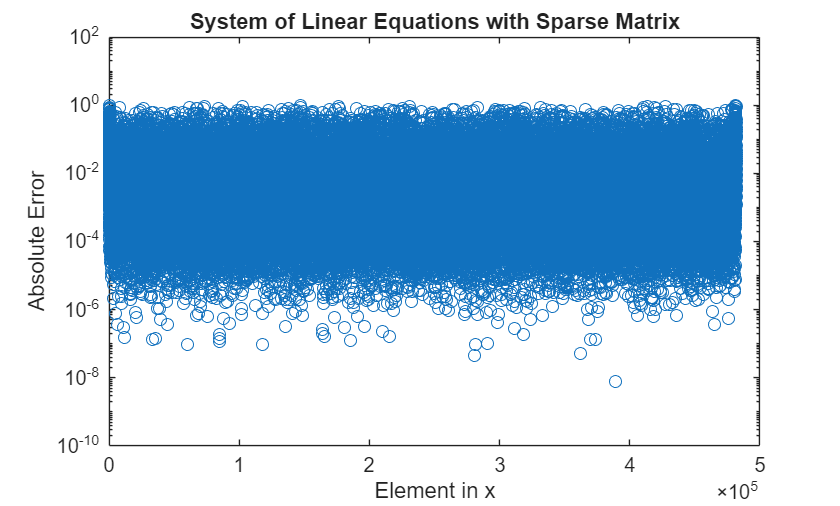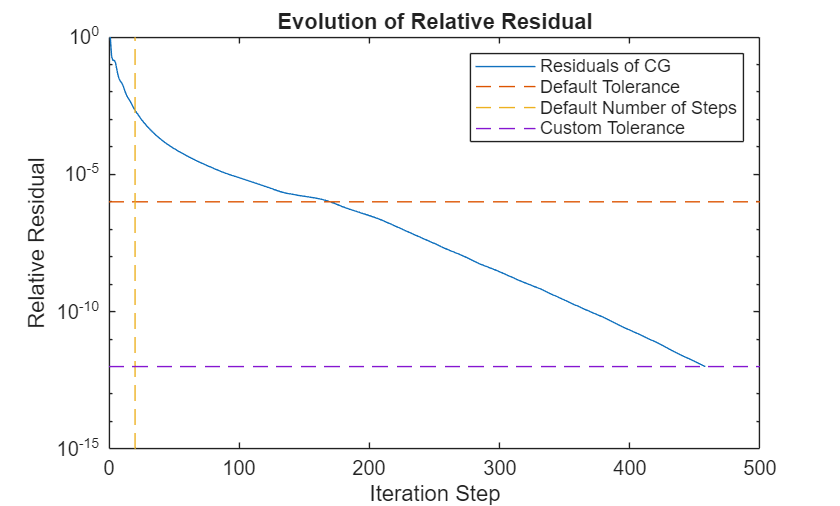# Use Distributed Arrays to Solve Systems of Linear Equations with Iterative Methods

For large-scale mathematical computations, iterative methods can be more efficient than direct methods. This example shows how you can solve systems of linear equations of the form $Ax=b$ in parallel using distributed arrays with iterative methods.

This example continues the topics covered in Use Distributed Arrays to Solve Systems of Linear Equations with Direct Methods. The direct solver methods implemented in `mldivide` can be used to solve distributed systems of linear equations in parallel but may not be efficient for certain large and sparse systems. Iterative methods generate a series of solutions from an initial guess, converging to a final result after several steps. These steps can be less computationally intensive than calculating the solution directly.

Distributed arrays distribute data from your client workspace to a parallel pool in your local machine or in a cluster. Each worker stores a portion of the array in its memory, but can also communicate with the other workers to access all segments of the array. Distributed arrays can contain different types of data including full and sparse matrices.

This example uses the `pcg` function to demonstrate how to solve large systems of linear equations using the conjugate gradient and the preconditioned conjugate gradient methods. Iterative methods can be used with both dense and sparse matrices but are most efficient for sparse matrix systems.

### Define your System of Linear Equations using a Sparse Matrix

When you use the Creation function, the software automatically starts a parallel pool using your default cluster settings. This example uses the Wathen matrix from the `gallery` function. This matrix is a sparse, symmetric, and random matrix with overall dimension $N=3{n}^{2}+4n+1$.

```n = 400; A = distributed(gallery('wathen',n,n));```
```Starting parallel pool (parpool) using the 'Processes' profile ... Connected to parallel pool with 6 workers. ```
`N = 3*n^2+4*n+1`
```N = 481601 ```

You can now define the right hand vector $b$. In this example, $b$ is defined as the row sum of $A$, which leads to an exact solution to $Ax=b$ of the form ${x}_{\text{exact}}=\left[1,...,1{\right]}^{T}$.

`b = sum(A,2);`

Since `sum` acts on a distributed array, `b` is also distributed and its data is stored in the memory of the workers of your parallel pool. Finally, you can define the exact solution for comparison with the solutions obtained using iterative methods.

`xExact = ones(N,1,'distributed');`

### Solve your System of Linear Equations with the Conjugate Gradient Method

The `pcg` MATLAB function provides the conjugate gradient (CG) method, which iteratively generates a series of approximate solutions for $\mathit{x}$, improving the solution with each step.

`[xCG_1,flagCG_1,relres_CG1,iterCG_1,resvecCG_1] = pcg(A,b);`

When the system is solved, you can check the error between each element of the obtained result `xCG_1` and the expected values of `xExact`. The error in the computed result is relatively high.

```errCG_1 = abs(xExact-xCG_1); figure(1) hold off semilogy(errCG_1,'o'); title('System of Linear Equations with Sparse Matrix'); ylabel('Absolute Error'); xlabel('Element in x');```The iterative computation ends when the series of approximate solutions converges to a specific tolerance or after the maximum number of iteration steps. For both distributed and on-client arrays, $pcg$ uses the same default settings:

• The default maximum tolerance is ${10}^{-6}$.

• The default maximum number of iteration steps is 20 or the order of coefficient matrix `A` if less than 20.

As a second output argument, the `pcg` function also returns a convergence flag that gives you more information about the obtained result, including whether the computed solution converged to the desired tolerance. For example, a value of `0` indicates the solution has properly converged.

`flagCG_1`
```flagCG_1 = 1 ```

In this example, the solution does not converge within the default maximum number of iterations, which results in the high error.

To increase the likelihood of convergence, you can customize the settings for tolerance and maximum number of iteration steps.

```tolerance = 1e-12; maxit = N; tCG = tic; [xCG_2,flagCG_2,relresCG_2,iterCG_2,resvecCG_2] = pcg(A,b,tolerance,maxit); tCG = toc(tCG); flagCG_2```
```flagCG_2 = 0 ```

With the custom settings, the solution converges. This solution has an improved absolute error compared to the previous solution.

```errCG_2 = abs(xExact-xCG_2); figure(2) hold off semilogy(errCG_1,'o'); hold on semilogy(errCG_2,'d'); title('Comparison of Absolute Error'); ylabel('Absolute Error'); xlabel('Element in x'); legend('Default tolerance and iterations','Improved tolerance and iterations'); hold off```The `pcg` method also returns a vector of the residual norm at each iteration step, `norm(b-A*x)/norm(b)`. The relative residual norm shows the ratio of accuracies between consecutive iteration steps. The evolution of the residuals during the iterative process can help you understand why the solution did not converge without custom settings.

```figure(3) f=semilogy(resvecCG_2./resvecCG_2(1)); hold on semilogy(f.Parent.XLim,[1e-6 1e-6],'--') semilogy([20 20], f.Parent.YLim,'--') semilogy(f.Parent.XLim,[1e-12 1e-12],'--') title('Evolution of Relative Residual'); ylabel('Relative Residual'); xlabel('Iteration Step'); legend('Residuals of CG','Default Tolerance','Default Number of Steps','Custom Tolerance') hold off```It is clear that the default number of steps is not enough to achieve a good solution for this system.

### Solve your System of Linear Equations with the Preconditioned Conjugate Gradient Method

You can improve the efficiency of solving your system using the preconditioned conjugate gradient (PCG) method. First, precondition your system of linear equations using a preconditioner matrix $\mathit{M}$. Next, solve your preconditioned system using the CG method. The PCG method can take much fewer iterations than the CG method.

The `pcg` function is also used for the PCG method. You can supply a suitable preconditioner matrix $\mathit{M}$as an additional input.

An ideal preconditioner matrix is a matrix whose inverse ${\mathit{M}}^{-1}$ is a close approximation to the inverse of the coefficient matrix, ${\mathit{A}}^{-1}$, but is easier to compute. This example uses the diagonal of $A$ to precondition the system of linear equations.

```M = spdiags(spdiags(A,0),0,N,N); tPCG = tic; [xPCG,flagPCG,relresPCG,iterPCG,resvecPCG]=pcg(A,b,tolerance,maxit,M); tPCG = toc(tPCG); figure(4) hold off;``````semilogy(resvecCG_2./resvecCG_2(1)) hold on; semilogy(resvecPCG./resvecPCG(1)) title('Evolution of Relative Residual'); ylabel('Relative Residual'); xlabel('Iteration Step'); legend('Residuals of CG','Residuals of PCG with M \approx diag(A)')```The previous figure shows that the PCG method needs drastically fewer steps to converge compared to the nonpreconditioned system. This result is also reflected in the execution times.

```fprintf([... '\nTime to solve system with CG: %d s', ... '\nTime to solve system with PCG: %d s'],tCG,tPCG);```
```Time to solve system with CG: 9.616080e+00 s Time to solve system with PCG: 1.399194e+00 s ```

As well as solving this example system in fewer iteration steps, the PCG method also returns a more accurate solution.

```errPCG = abs(xExact-xPCG); figure(5) hold off semilogy(errCG_1,'o'); hold on semilogy(errCG_2,'d'); semilogy(errPCG,'x'); title('Comparison of absolute error'); ylabel('Absolute error'); xlabel('Element in x'); legend('CG default','CG custom','PCG');```After you are done with your computations, you can delete your parallel pool. The `gcp` function returns the current parallel pool object so you can delete the current pool.

`delete(gcp('nocreate'))`

The Wathen matrix used in this example is a good demonstration of how a good preconditioner can dramatically improve the efficiency of the solution. The Wathen matrix has relatively small off-diagonal components, so choosing$M=\text{diag}\left(A\right)$ gives a suitable preconditioner. For an arbitrary matrix $\mathit{A}$, finding a preconditioner might not be so straightforward.

For an example of how to approximate a differential equation by a linear system and solve it using a distributed iterative solver with a multigrid preconditioner, see Solve Differential Equation Using Multigrid Preconditioner on Distributed Discretization.